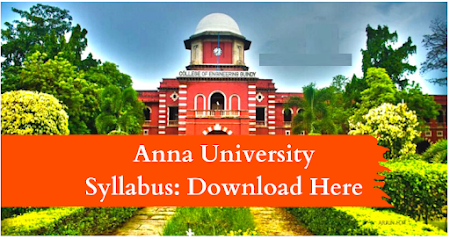### MA8402    PROBABILITY AND QUEUING THEORY                 L    T    P    C        4    0    0     4

OBJECTIVES:  To provide necessary basic concepts in probability and random processes for applications     such as random signals, linear systems in communication engineering.  To understand the basic concepts of probability, one and two dimensional random variables and to introduce some standard distributions applicable to engineering which can describe real life phenomenon.   To understand the basic concepts of random processes which are widely used in IT fields.  To understand the concept of queueing  models and apply in engineering.  To understand the significance of advanced queueing  models.   To provide the required mathematical support in real life problems and develop probabilistic models which can be used in several areas of science and engineering.

UNIT I  PROBABILITY AND RANDOM   VARIABLES                                                          12 Probability – Axioms of probability – Conditional probability – Baye‘s theorem - Discrete and continuous random variables – Moments – Moment generating functions – Binomial, Poisson,  Geometric, Uniform, Exponential  and Normal distributions.

UNIT II  TWO - DIMENSIONAL   RANDOM   VARIABLES                                                   12 Joint distributions – Marginal and conditional distributions – Covariance – Correlation and linear regression – Transformation of random variables – Central limit theorem (for independent and identically distributed random variables).

UNIT III          RANDOM PROCESSES                                                                                        12 Classification – Stationary process – Markov process - Poisson process – Discrete parameter Markov chain – Chapman Kolmogorov equations – Limiting distributions.

UNIT IV        QUEUEING MODELS                                                                                             12 Markovian queues – Birth and death processes – Single and multiple server queueing models – Little‘s formula - Queues with finite waiting rooms – Queues with impatient customers : Balking and reneging.   UNIT V  ADVANCED QUEUEING MODELS 12 Finite source models - M/G/1 queue – Pollaczek Khinchin formula  - M/D/1 and M/EK/1 as special cases –   Series queues – Open Jackson networks.

TOTAL :  60 PERIODS
OUTCOMES: Upon successful completion of the course, students should be able to:    Understand the fundamental knowledge of the concepts of probability and have knowledge   of  standard distributions which can describe real life phenomenon.    Understand the basic concepts of one and two dimensional random variables and apply in   engineering  applications.  Apply the concept of random processes in engineering disciplines.      Acquire skills in analyzing queueing models.    Understand and characterize phenomenon which evolve with respect to time  in a          probabilistic manner

TEXTBOOKS:
1. Gross, D., Shortle, J.F, Thompson, J.M and Harris. C.M., ―Fundamentals of Queueing Theory", Wiley Student 4th Edition, 2014.
2. Ibe, O.C., ―Fundamentals of Applied Probability and Random Processes", Elsevier, 1st Indian Reprint, 2007.

REFERENCES :
1. Hwei Hsu, "Schaum‘s Outline of Theory and Problems of Probability, Random Variables and Random Processes", Tata McGraw Hill Edition, New Delhi, 2004.
2. Taha, H.A., "Operations Research", 9th Edition, Pearson India Education Services, Delhi, 2016.
3. Trivedi, K.S., "Probability and Statistics with Reliability, Queueing and Computer Science Applications", 2nd Edition, John Wiley and Sons, 2002.
4. Yates, R.D. and Goodman. D. J., "Probability and Stochastic Processes", 2nd Edition, Wiley India Pvt. Ltd., Bangalore, 2012.